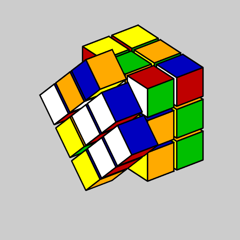# Qube, The Movie### Contents

#### The Movie

Here is a link to "Qube, The Movie", a video made with Qube, my digital Rubik's cube simulator. Mathematically, all of the action is driven by the 3-by-3 matrices on display.

#### Scaling

Qube uses a 3x3x3 array of identical cubelets . The coordinates [x,y,z] of the centers of the cubelets are all of the possible combinations of -2, 0 and +2. The vertices of an individual cubelet form a 8-by-3 matrix. The width of a cubelet is controlled by multiplying its vertices by a 3-by-3 diagonal scaling matrix.

Our video begins with the width near zero, so the cubelets appear to be points at the centers. The width is increased to a value where the cubelets almost touch each other. The small gap is maintained for its visual effect.

#### Rubik's Rotations

The primary operations with a Rubik's cube are the simultaneous rotation of all the cubelets contained in one of the six faces. For example, this video shows a slow-motion rotation of the "Left" face about the x-axis.

It is also possible to rotate the three interior "slices", as well as the entire cube about one of the coordinate axes.

#### Types

The type of a cubelet is the number of nonzeros in the coordinates of its center.

  type = nnz([x,y,z])

The cubelet in the center of the puzzle has type = 0 and the six cubelets with type = 1 are located at the center of each face. We use 0:1 to denote the set of these seven cubelets. In a real, physical Rubick's Cube, 0:1 is a single solid central core that holds the entire puzzle together.

There are twelve type = 2 cubelets located on the edges of each face and eight type = 3 cubelets at the corners of the puzzle. So, 0:3 denotes the entire cube and 2:3 is the corners and edges without the central core. Qube, The Movie shows the types in this order:

  0:3, 0:2, 0:1, 0, 1, 2, 3, 2:3

Careful readers of this blog should recognize 2:3 as level one of the Menger sponge fractal.

Published with MATLAB® R2022a

|